# Four Fundamental Forces Gravity Attractive only Property of

• Slides: 36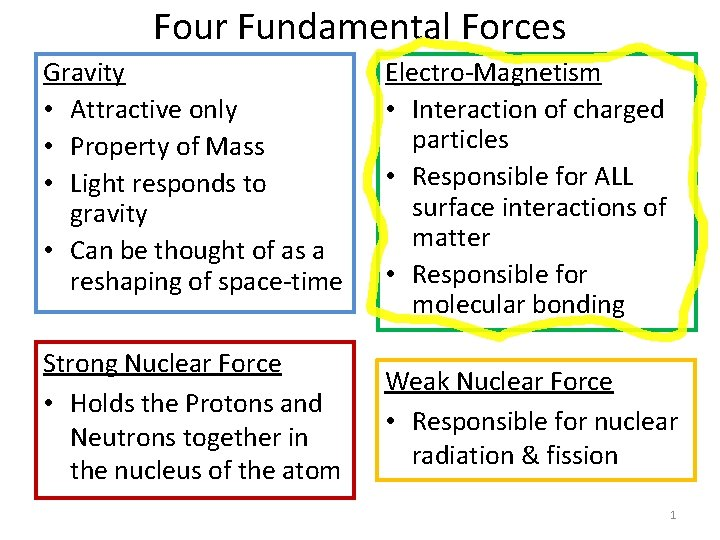Four Fundamental Forces Gravity • Attractive only • Property of Mass • Light responds to gravity • Can be thought of as a reshaping of space-time Electro-Magnetism • Interaction of charged particles • Responsible for ALL surface interactions of matter • Responsible for molecular bonding Strong Nuclear Force • Holds the Protons and Neutrons together in the nucleus of the atom Weak Nuclear Force • Responsible for nuclear radiation & fission 1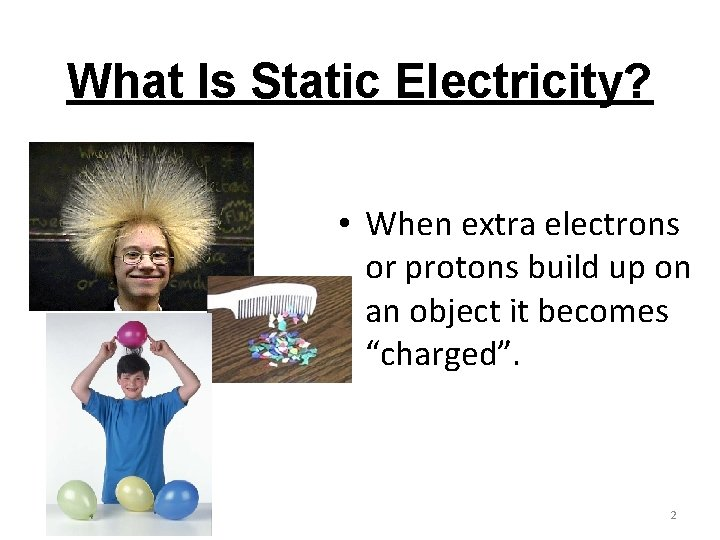What Is Static Electricity? • When extra electrons or protons build up on an object it becomes “charged”. 2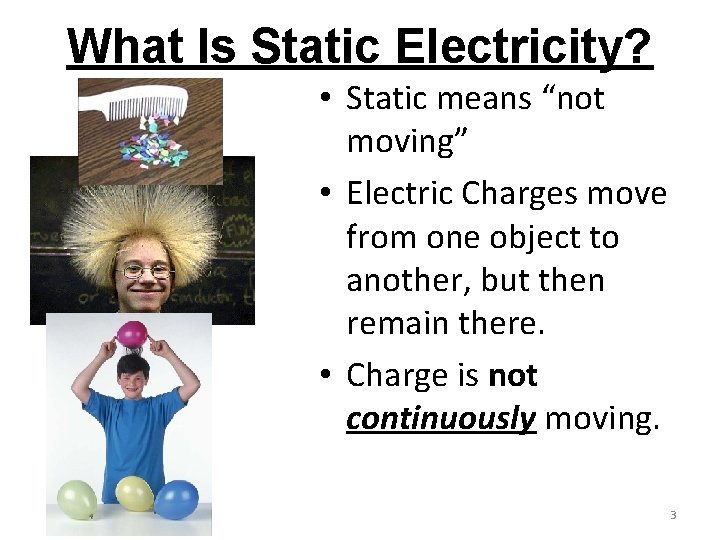What Is Static Electricity? • Static means “not moving” • Electric Charges move from one object to another, but then remain there. • Charge is not continuously moving. 3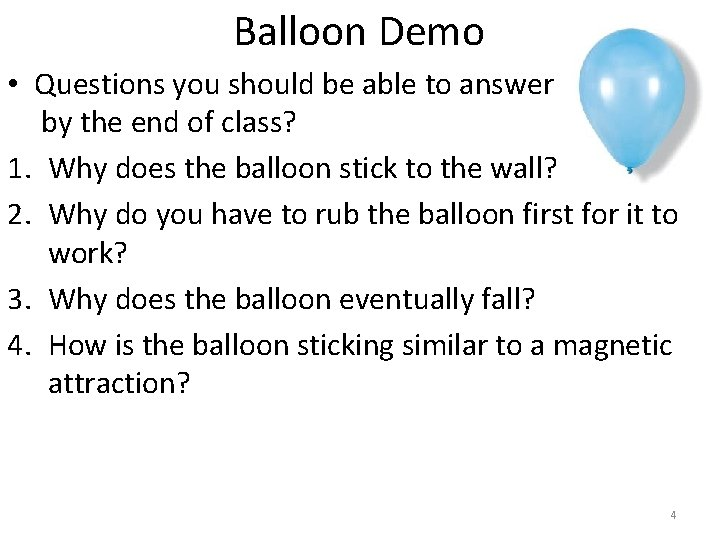Balloon Demo • Questions you should be able to answer by the end of class? 1. Why does the balloon stick to the wall? 2. Why do you have to rub the balloon first for it to work? 3. Why does the balloon eventually fall? 4. How is the balloon sticking similar to a magnetic attraction? 4Where do charges come from? All charges come from atoms. All we can ever do is transfer electrons from one object to another. – Charge is a CONSERVED quantity. We CAN NOT make (or destroy) charge!** **When an electron is created in a particle accelerator, and antielectron also forms, so that the net charge remains = 0. 5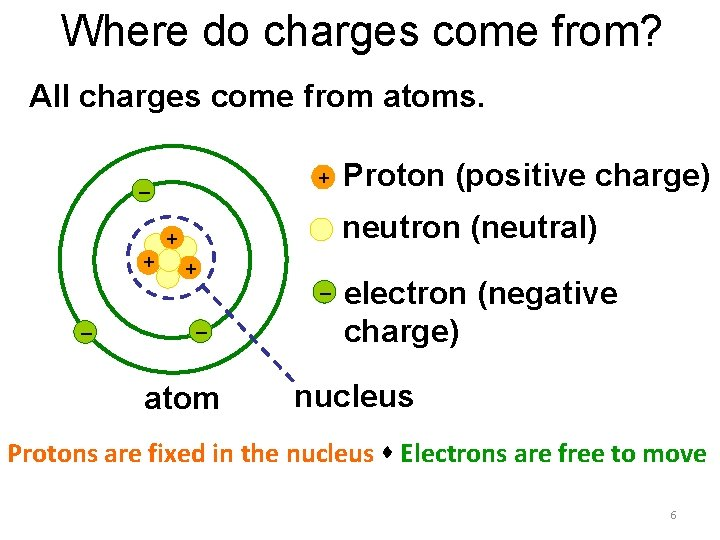Where do charges come from? All charges come from atoms. + – neutron (neutral) + + + – – Proton (positive charge) – atom electron (negative charge) nucleus Protons are fixed in the nucleus Electrons are free to move 6Electrical Charge, q • Charge of an electron: qe = 1. 60 x 10 -19 C • Unit: Coulomb (C) • Charge of an Proton: +1. 6 x 10 -19 C • 1 Coulomb = 6. 25 x 1018 protons • 1 Coulomb = 6. 25 x 1018 electrons 7Practice Problem • 8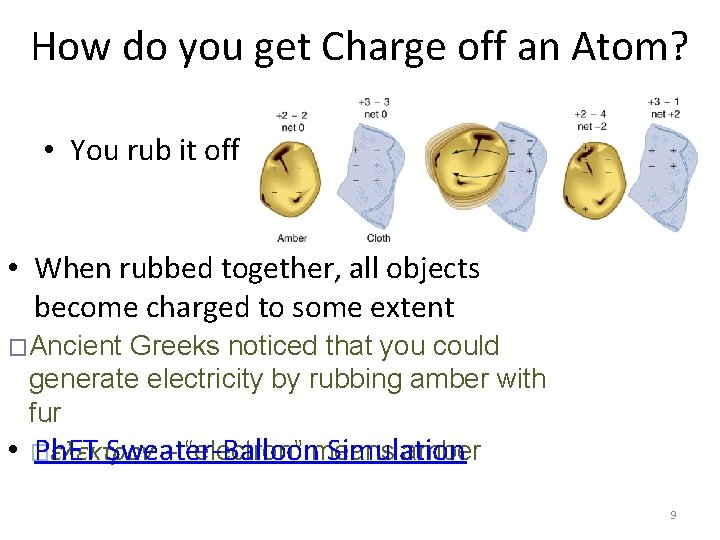How do you get Charge off an Atom? • You rub it off • When rubbed together, all objects become charged to some extent �Ancient Greeks noticed that you could generate electricity by rubbing amber with fur • � Ph. ET Sweater-Balloon Simulation ελεκτρον – “electron” means amber 9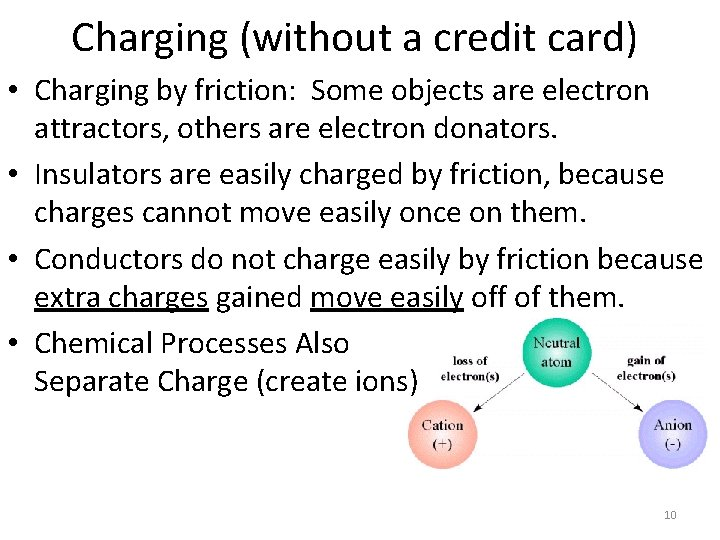Charging (without a credit card) • Charging by friction: Some objects are electron attractors, others are electron donators. • Insulators are easily charged by friction, because charges cannot move easily once on them. • Conductors do not charge easily by friction because extra charges gained move easily off of them. • Chemical Processes Also Separate Charge (create ions) 10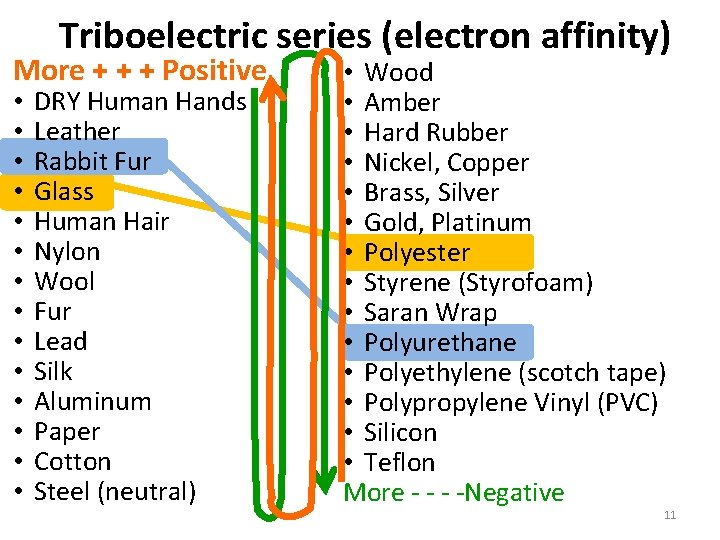Triboelectric series (electron affinity) More + + + Positive • • • • DRY Human Hands Leather Rabbit Fur Glass Human Hair Nylon Wool Fur Lead Silk Aluminum Paper Cotton Steel (neutral) • Wood • Amber • Hard Rubber • Nickel, Copper • Brass, Silver • Gold, Platinum • Polyester • Styrene (Styrofoam) • Saran Wrap • Polyurethane • Polyethylene (scotch tape) • Polypropylene Vinyl (PVC) • Silicon • Teflon More - -Negative 11Three Charge States neutral # electron = # protons Positive # electrons < # protons (electrons lost) negative # electrons > # protons (electrons gained) 12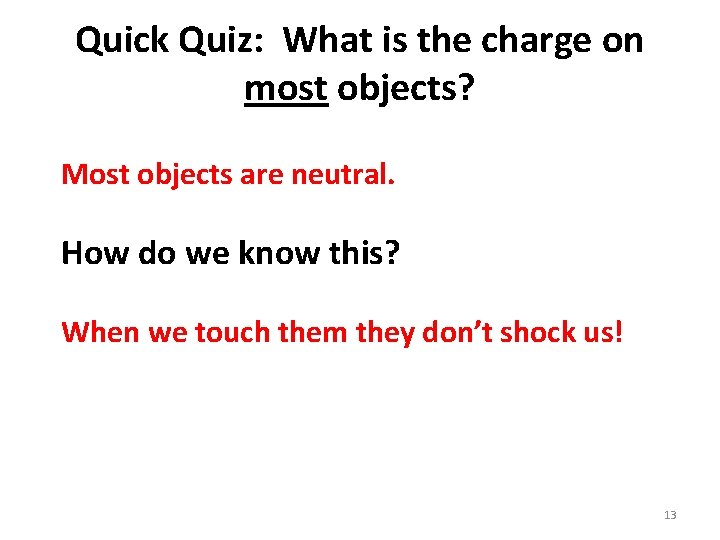Quick Quiz: What is the charge on most objects? Most objects are neutral. How do we know this? When we touch them they don’t shock us! 13What Holds the Balloon to the Wall? and stops if from falling? There must be a FORCE Coulomb’s Force! 14Electric Force: Coulomb’s Law • Originally derived by 18 th Century French physicist Charles Coulomb • Describes interaction force for electrically charged objects • Experimentally verified in modern times • No exceptions found so far (even at distances within the atom) 15Electric Force: Coulomb’s Law Electric Force UNITS: Newtons (N) q = first point charge in Coulombs (C) Q = second point charge in Coulombs (C) r = distance between q & Q k = constant 8. 988 x 109 or 8. 99 x 109 16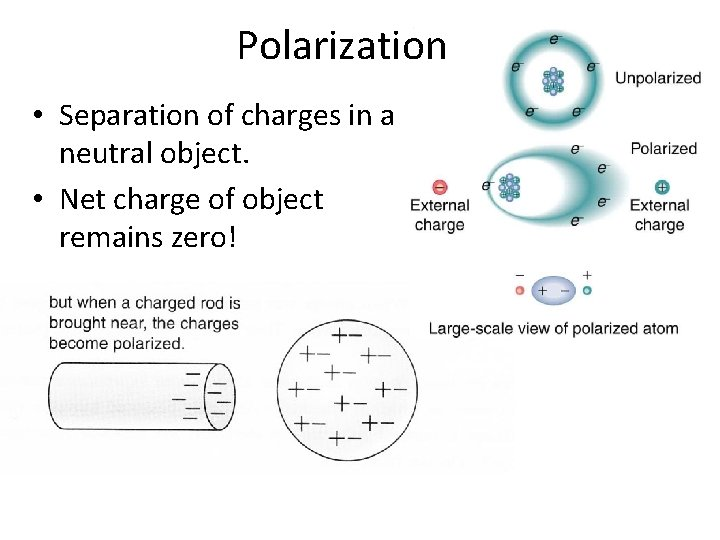Polarization • Separation of charges in a neutral object. • Net charge of object remains zero!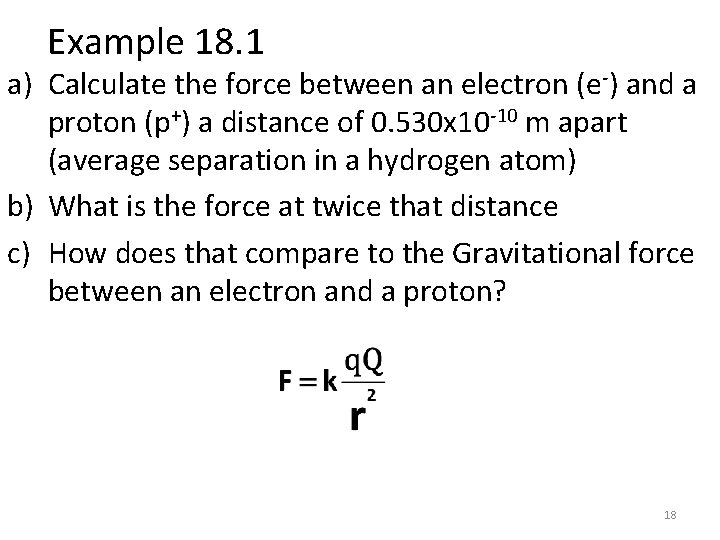Example 18. 1 a) Calculate the force between an electron (e-) and a proton (p+) a distance of 0. 530 x 10 -10 m apart (average separation in a hydrogen atom) b) What is the force at twice that distance c) How does that compare to the Gravitational force between an electron and a proton? 18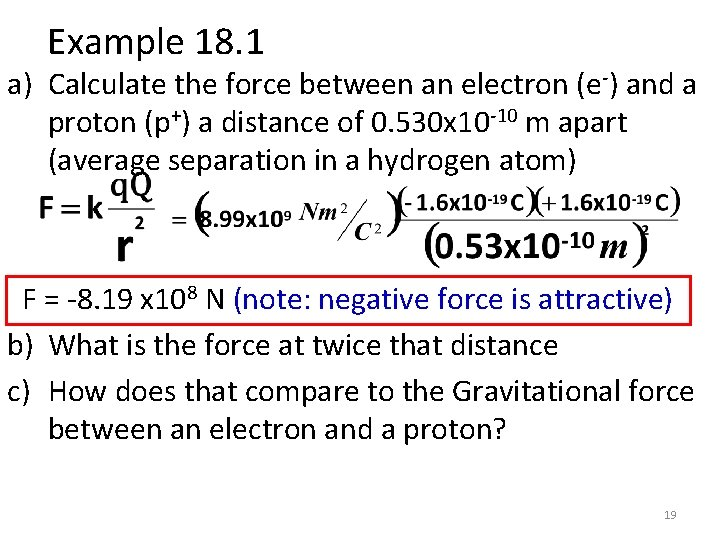Example 18. 1 a) Calculate the force between an electron (e-) and a proton (p+) a distance of 0. 530 x 10 -10 m apart (average separation in a hydrogen atom) F = -8. 19 x 108 N (note: negative force is attractive) b) What is the force at twice that distance c) How does that compare to the Gravitational force between an electron and a proton? 19Example 18. 1 a) Calculate the force between an electron (e-) and a proton (p+) a distance of 0. 530 x 10 -10 m apart (average separation in a hydrogen atom) F = -8. 19 x 10 -8 N (note: negative force is attractive) b) What is the force at twice that distance F = -2. 05 x 10 -8 N [note: ¼ of the force in (a)] c) How does that compare to the Gravitational force between an electron and a proton? 20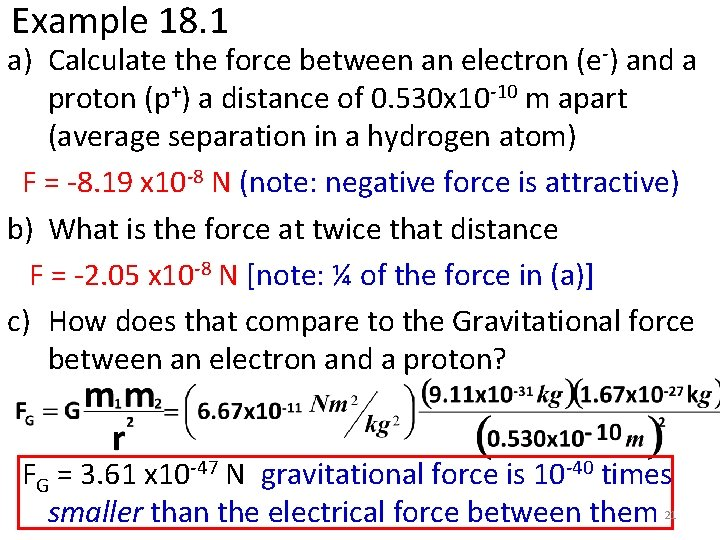Example 18. 1 a) Calculate the force between an electron (e-) and a proton (p+) a distance of 0. 530 x 10 -10 m apart (average separation in a hydrogen atom) F = -8. 19 x 10 -8 N (note: negative force is attractive) b) What is the force at twice that distance F = -2. 05 x 10 -8 N [note: ¼ of the force in (a)] c) How does that compare to the Gravitational force between an electron and a proton? FG = 3. 61 x 10 -47 N gravitational force is 10 -40 times smaller than the electrical force between them 21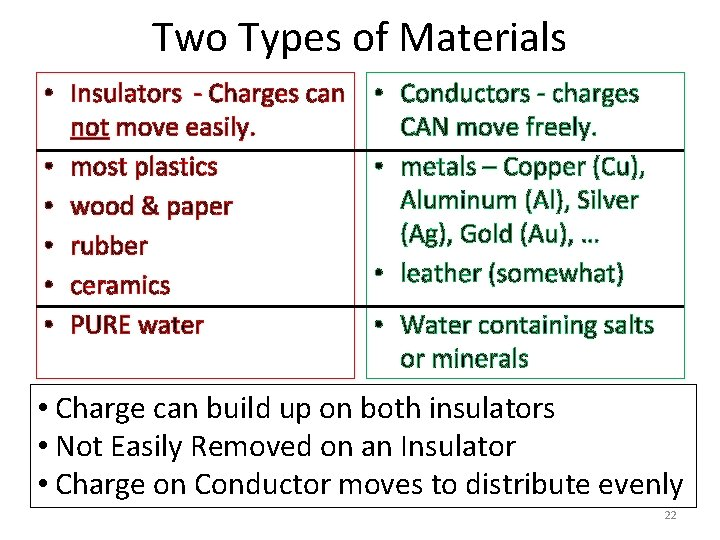Two Types of Materials • Insulators - Charges can not move easily. • most plastics • wood & paper • rubber • ceramics • PURE water • Conductors - charges CAN move freely. • metals – Copper (Cu), Aluminum (Al), Silver (Ag), Gold (Au), … • leather (somewhat) • Water containing salts or minerals • Charge can build up on both insulators • Not Easily Removed on an Insulator • Charge on Conductor moves to distribute evenly 22Are YOU an insulator or a conductor? • Conductor … why? • You are about 80% salty water. • Electrons are mostly free to move on your sweaty skin (and into your wet, salty body). 23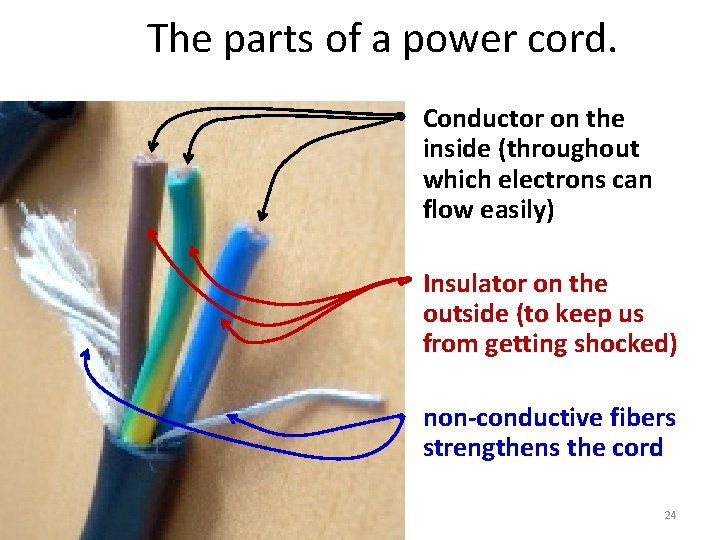The parts of a power cord. • Conductor on the inside (throughout which electrons can flow easily) • Insulator on the outside (to keep us from getting shocked) • non-conductive fibers strengthens the cord 24Electrostatic Repulsion & Attraction • 2 Types of Charge: (+) and ( ) • Like Charges Repel +- +- • Opposite Charges Attract + - 25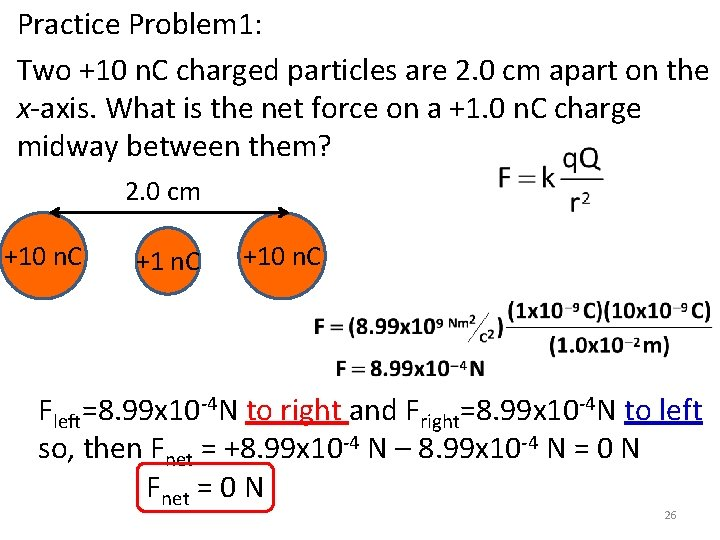Practice Problem 1: Two +10 n. C charged particles are 2. 0 cm apart on the x-axis. What is the net force on a +1. 0 n. C charge midway between them? 2. 0 cm +10 n. C Fleft=8. 99 x 10 -4 N to right and Fright=8. 99 x 10 -4 N to left so, then Fnet = +8. 99 x 10 -4 N – 8. 99 x 10 -4 N = 0 N Fnet = 0 N 26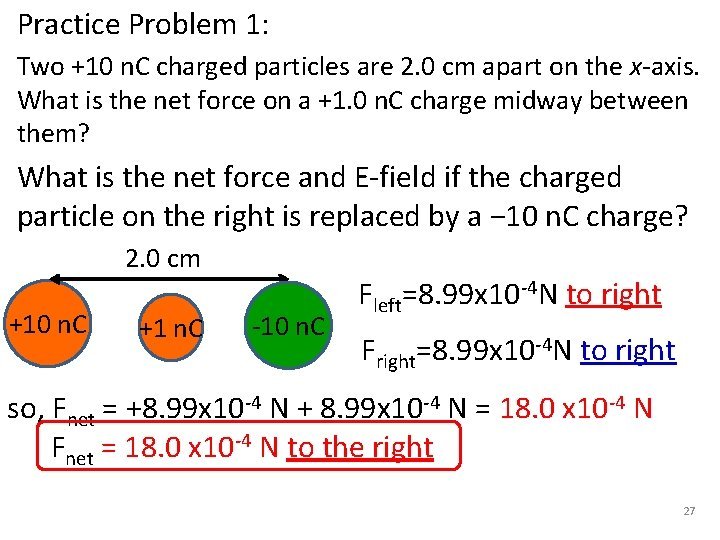Practice Problem 1: Two +10 n. C charged particles are 2. 0 cm apart on the x-axis. What is the net force on a +1. 0 n. C charge midway between them? What is the net force and E-field if the charged particle on the right is replaced by a − 10 n. C charge? 2. 0 cm +10 n. C +1 n. C -10 n. C Fleft=8. 99 x 10 -4 N to right Fright=8. 99 x 10 -4 N to right so, Fnet = +8. 99 x 10 -4 N + 8. 99 x 10 -4 N = 18. 0 x 10 -4 N Fnet = 18. 0 x 10 -4 N to the right 27Electric Force & Electric Field • The force on the test charge according to Coulomb’s Law for a charge Q and test charge q • Electric Field, E (units N/C) E-field at a distance, r , away from charge Q. 28What is the E-field for Practice Problem 1: Two +10 n. C charged particles are 2. 0 cm apart on the x-axis. What is the net force on a +1. 0 n. C charge midway between them? What is the E-field at this point? 2. 0 cm +10 n. C Fnet = 0 N Enet=Fnet/q where q is the 1 n. C charge. Enet=0 N/C 29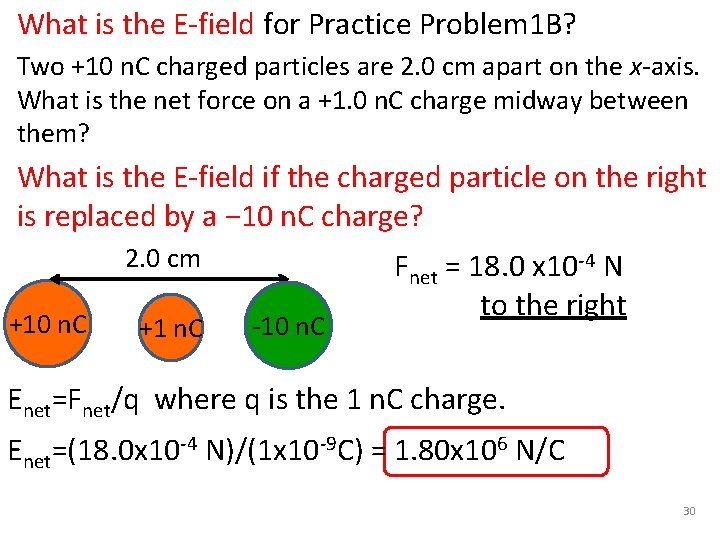What is the E-field for Practice Problem 1 B? Two +10 n. C charged particles are 2. 0 cm apart on the x-axis. What is the net force on a +1. 0 n. C charge midway between them? What is the E-field if the charged particle on the right is replaced by a − 10 n. C charge? 2. 0 cm +10 n. C +1 n. C -10 n. C Fnet = 18. 0 x 10 -4 N to the right Enet=Fnet/q where q is the 1 n. C charge. Enet=(18. 0 x 10 -4 N)/(1 x 10 -9 C) = 1. 80 x 106 N/C 30Every Charge Generates an Electric Field • A positive test charge, q, will move in the direction of the field arrows. • A NEGATIVE charge, -q, will move OPPOSITE the direction of the field arrows • Charges move because the electric field induces a FORCE to accelerate them. + +q -q 31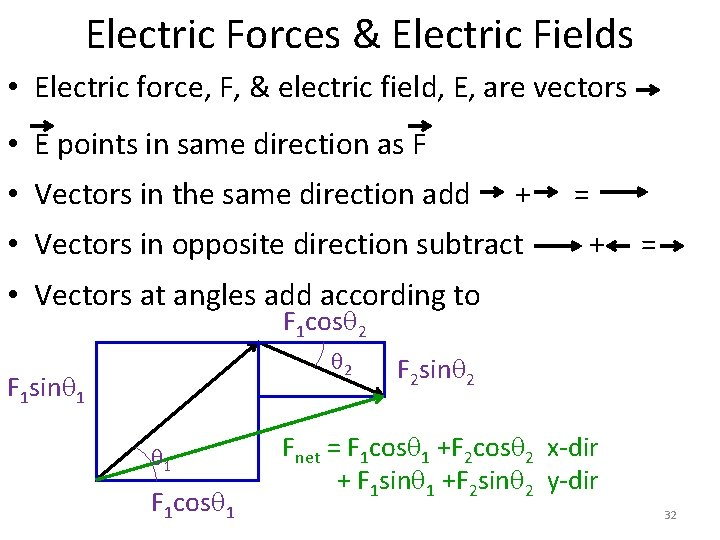Electric Forces & Electric Fields • Electric force, F, & electric field, E, are vectors • E points in same direction as F • Vectors in the same direction add + = • Vectors in opposite direction subtract + = • Vectors at angles add according to F 1 cos 2 2 F 1 sin 1 1 F 1 cos 1 F 2 sin 2 Fnet = F 1 cos 1 +F 2 cos 2 x-dir + F 1 sin 1 +F 2 sin 2 y-dir 32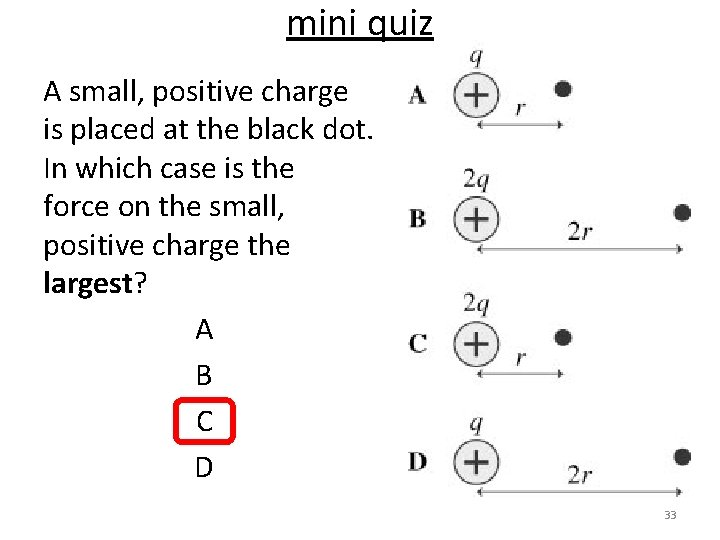mini quiz A small, positive charge is placed at the black dot. In which case is the force on the small, positive charge the largest? A B C D 33mini quiz All charges in the diagrams are equal magnitude. In each case, a small positive charge is placed at the black dot. In which cases is the force on this charge: 1. to the right? 2. to the left? 3. zero? 34Sample Problem- Three point charges of magnitude +1 C, +1 C and − 1 C respectively are placed on the three corners of an equilateral triangle as shown. Which vector best represents the direction of the net force acting on the − 1 C charge as a result of the forces exerted by the other two charges? - + Fnet + a) b) c) d)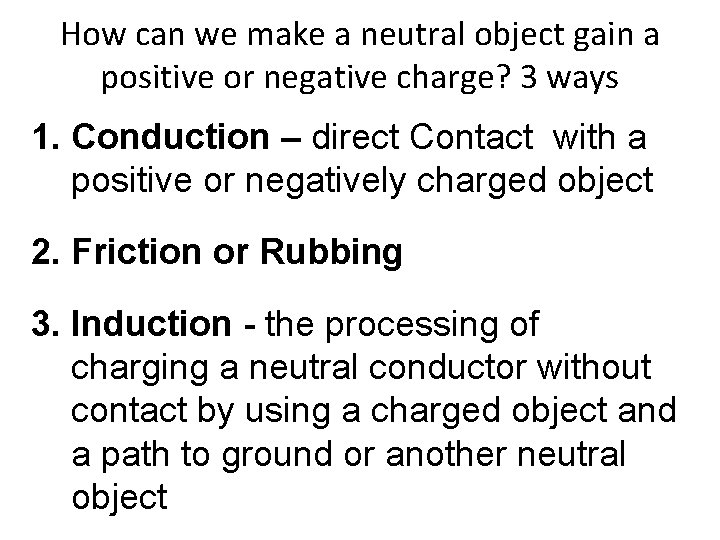How can we make a neutral object gain a positive or negative charge? 3 ways 1. Conduction – direct Contact with a positive or negatively charged object 2. Friction or Rubbing 3. Induction - the processing of charging a neutral conductor without contact by using a charged object and a path to ground or another neutral object